# Civil Engineering - Strength of Materials

### Exercise :: Strength of Materials - Section 1

11.

A uniform girder simply supported at its ends is subjected to a uniformly distributed load over its entire length and is propped at the centre so as to neutralise the deflection. The net B.M. at the centre will be

 A. WL B.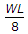C.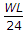D.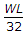E.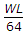Explanation:

No answer description available for this question. Let us discuss.

12.

A beam of length L is pinned at both ends and is subjected to a concentrated bending couple of moment M at its centre. The maximum bending moment in the beam is

 A. M B. M/2 C. M/3 D. ML/2

Explanation:

No answer description available for this question. Let us discuss.

13.

If two forces acting at a joint are not along the straight line, then for the equilibrium of the joint

 A. one of the forces must be zero B. each force must be zero C. forces must be equal and of the same sign D. forces must be equal in magnitude but opposite in sign.

Explanation:

No answer description available for this question. Let us discuss.

14.

A closely coiled helical spring of radius R, contains n turns and is subjected to an axial load W. If the radius of the coil wire is r and modulus of rigidity of the coil material is C, the stress developed in the helical spring is

 A.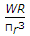B.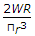C.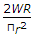D.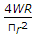Explanation:

No answer description available for this question. Let us discuss.

15.

If the shear force along a section of a beam is zero, the bending moment at the section is

 A. zero B. maximum C. minimum D. average of maximum-minimum E. none of these.